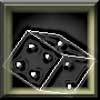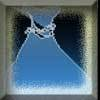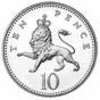#### You may also like### Gambling at Monte Carlo

A man went to Monte Carlo to try and make his fortune. Is his strategy a winning one?### Marbles and Bags

Two bags contain different numbers of red and blue marbles. A marble is removed from one of the bags. The marble is blue. What is the probability that it was removed from bag A?### Coin Tossing Games

You and I play a game involving successive throws of a fair coin. Suppose I pick HH and you pick TH. The coin is thrown repeatedly until we see either two heads in a row (I win) or a tail followed by a head (you win). What is the probability that you win?

# Chances Are

##### Age 14 to 16Challenge Level

Rajeev from Fair Field School sent us the following solution:
I found that your best chance of winning was the option of tossing the coin and the chance of winning was $\frac{1}{4096}$.
Here is how I worked it out:
1. You win first prize if you can toss a fair coin and get 12 heads in a row. With one coin toss, you get half a chance, with 2 coin tosses you get $\frac{1}{4}$ and with 3 you get $\frac{1}{8}$ so with 12 coin tosses you get$(\frac{1}{2})^{12}$ which is $\frac{1}{4096}$
2. Throw 5 fair dice and you get 5 sixes and you win the first prize. With 1 fair die your chance of getting a six is $\frac{1}{6}$ and with 2 its $\frac{1}{36}$ so with 5 fair dice its $(\frac{1}{6})^5$ which is $\frac{1}{7776}$
3. Choose the top 4 from 10 famous pictures and put them in the right order to win. $\frac{1}{5040}$
4. Our resident Gardener has listed her seven favourite plants in order. If you can match the order you win. With 2 there are 2 ways of ordering, with 3 it is 6 and with 4 it is 24 and so with 7 it is $7\times6\times5\times4\times3\times2\times1)=5040$ So the probability of selecting the correct ordering is $\frac{1}{5040}$
5. You toss four ten-sided dice and win the first prize if you can get 4 sixes. With one die it's $\frac{1}{10}$ and with 2 dice it's $\frac{1}{100}$ and with 4 dice it is $\frac{1}{10000}$
Christian from Takeley explains how to calculate the probability for number 3:
The game which involves the ten famous pictures. You work out the probability of putting the right picture first, which is one tenth. You then work out the probability of getting the right picture and putting it into the second place, which is one ninth. You continue in this way until you have all four individual probabilities. You then multiply them all together: one tenth, one ninth, one eight and one seventh, to get your final answer. The probability is $\frac{1}{5040}$, which by coincidence is the same probability as in the gardener game.

Well done too to Patrick from Woodbridge School and Yash from Natomas Charter School who sent in largely complete solutions to this problem. Yash commented:
Since all the numerators are the same, the greatest probability would be have to be the least denominator. Thus you'd be best off tossing a coin 12 times, but it's probably not as much fun.

And Patrick pointed out:
Thus, the best deal seems to be the twelve heads in a row - which is so unlikely that it would be better to buy that bottle of water instead!